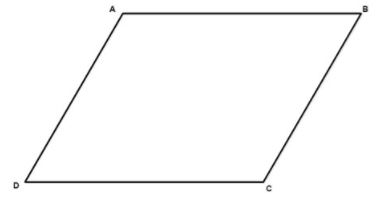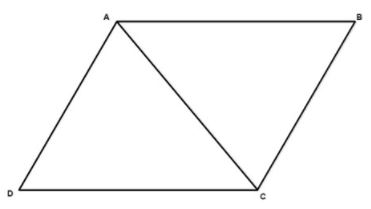Courses

# Theory - To explore Similarities & Differences in properties of the Quadrilaterals, Class 9 Math Class 9 Notes | EduRev

## Mathematics (Maths) Class 9

Created by: Indu Gupta

## Class 9 : Theory - To explore Similarities & Differences in properties of the Quadrilaterals, Class 9 Math Class 9 Notes | EduRev

The document Theory - To explore Similarities & Differences in properties of the Quadrilaterals, Class 9 Math Class 9 Notes | EduRev is a part of the Class 9 Course Mathematics (Maths) Class 9.
All you need of Class 9 at this link: Class 9

Objective :

To explore similarities and differences in the properties with respect to diagonals of the following quadrilaterals- a parallelogram, a square, a rectangle and a rhombus.

Definition:

It is a quadrilateral where both pairs of opposite sides are parallel. Quadrilateral ABCD is a parallelogram.Properties :

• A diagonal of a parallelogram divides it into two congruent triangles (▲ ADB is congruent to ▲ ABC).

• Diagonals of a parallelogram bisect each other.

• Opposite sides are congruent (AB = DC).

• Opposite angles are congruent (∠ADC= ∠ABC).

• Consecutive angles are supplementary (∠DAB + ∠ADC = 180°).

• If one angle is right angle, then all angles are right.## Mathematics (Maths) Class 9

192 videos|230 docs|82 tests

,

,

,

,

,

,

,

,

,

,

,

,

,

,

,

,

,

,

,

,

,

,

,

,

;